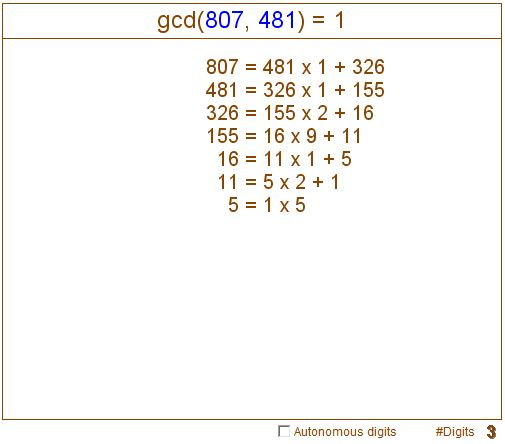Euclid's Algorithm

Euclid's algorithm is a famous procedure for finding the gcd, i.e., greatest common divisor (factor) of two integers. The idea is pretty simple. If N = M×s, with N, M, s, positive integers, then any divisor of M is also a divisor of N, making M their greatest common divisor:

If  N = M×s then M = gcd(N, M).

when N = M×s + R (where all four are positive integers), then every common divisor of M and N is also a divisor of R. Actually, any common divisor of any pair of these three numbers is a divisor of the third; so that, with R ≠ 0,

N = M×s + R implies gcd(N, M) = gcd(M, R).

Euclid's algorithm is based on the observation that taking R to be the remainder of the division of N by M makes R < M. Which means that the task of finding the gcd of N and M, with M < N, can be reduced to the same problem but with smaller numbers, M and R. Doing that we'll get another remainder even smaller than R and so on until the remainder becomes 0. This is assured since on every step we are dealing with smaller numbers, but a decreasing sequence of integers is necessarily finite. In the case of the remainders in Euclid's algorithm the last term must be 0 for, otherwise, one would be able to perform at least one more step of the algorithm.

The applet below illustrates Euclid's algorithm. The two numbers on top are modifiable digit-by-digit. Click a little off the vertical midline of the digit you wish to modify. (For a faster action just drag the mouse up and down.) When the box "Autonomous digits" is checked, trying to increase, say, 9 in 19 will result in 10, otherwise it will be 20. The number of digits is also controlled at the bottom of the applet.

If you are reading this, your browser is not set to run Java applets. Try IE11 or Safari and declare the site https:///www.cut-the-knot.org as trusted in the Java setup.What if applet does not run?